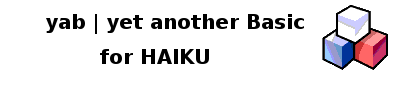Getting the system fonts - Printable Version +- yab | yet another Basic for HAIKU (http://yab.orgfree.com/forum) +-- Forum: Programming in yab (http://yab.orgfree.com/forum/forumdisplay.php?fid=1) +--- Forum: Snippets (http://yab.orgfree.com/forum/forumdisplay.php?fid=3) +--- Thread: Getting the system fonts (/showthread.php?tid=84) Getting the system fonts - bbjimmy - 03-21-2017 yab did not include a way to determine the currently set system font. this library file should do the trick! https://github.com/bbjimmy/yab-lib/tree/master/getsystemfonts Code:```//export sub getfont_family\$(fonttype\$) //returns the string needed by draw set to set this font -- less the point size. //export sub getfont_size\$(fonttype\$) // returns the piont size of the font //fonttype\$ can be "bold", "book", or "mono" //////////////////////////////////// sub getsystemfonts() local a\$,num,x dim font\$(1) a\$=system\$("message /boot/home/config/settings/system/app_server/fonts") num=split(a\$,font\$(),"\n") for x=2 to 10     a\$=gststring\$(font\$(x))     if a\$<>"" font\$(x)=a\$ next font\$(4)=getsize\$(font\$(4)) font\$(7)=getsize\$(font\$(7)) font\$(10)=getsize\$(font\$(10)) end sub //////////////////////////////////// sub gststring\$(a\$) local x x=instr(a\$,chr\$(34)) a\$=right\$(a\$,len(a\$)-x) x=instr(a\$,chr\$(34)) a\$=left\$(a\$,x-1) return a\$ end sub //////////////////////////////////// sub getsize\$(a\$) x=instr(a\$,"(") a\$=right\$(a\$,len(a\$)-x) x=instr(a\$,".") a\$=left\$(a\$,x-1) return a\$ end sub //////////////////////////////////// export sub getfont_family\$(fonttype\$) dim font\$(1) x=arraysize(font\$(),1) if x=1 getsystemfonts() fonttype\$=lower\$(fonttype\$) if fonttype\$="book" return font\$(2)+", "+font\$(3)+", " if fonttype\$="bold" return font\$(5)+", "+font\$(6)+", " if fonttype\$="mono" return font\$(8)+", "+font\$(9)+", " end sub //////////////////////////////////// export sub getfont_size\$(fonttype\$) dim font\$(1) x=arraysize(font\$(),1) if x=1 getsystemfonts() fonttype\$=lower\$(fonttype\$) if fonttype\$="book" return font\$(4) if fonttype\$="bold" return font\$(7) if fonttype\$="mono" return font\$(10) end sub``` RE: Getting the system fonts - bbjimmy - 04-23-2017 I added a fallback, in case there is no font settings file. The file is now hosted on github: https://github.com/bbjimmy/yab-lib